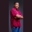Related Tags

typescript
array
slice
communitycreator

# What is array.slice() in TypeScript?Theodore Kelechukwu Onyejiaku

### Overview

TypeScript is a superset of JavaScript. Wherever JavaScript runs, TypeScript runs, too. We can use the slice() method in TypeScript. This method is used to extract a certain section or parts of the elements of an array.

### Syntax

array.slice(begin, end)
Syntax for slice() method in TypeScript

### Parameters

begin: This is the index position to start or begin the extraction. It is an integer value.

end: This is the index position to end the extraction. It has be an integer as well.

### Return value

The value returned is the part of the array array that was extracted.

### Example

// create arrays in TypeScript
let names: string[] = ["Theodore", "James", "Peter", "Amaka"]
let numbers : Array<number>;
numbers = [12, 34, 5, 0.9]
let cars : Array<string> = ["Porsche", "Toyota", "Lexus"]
let randomValues : Array<string | number> = ["one",1, "two", 2, 56]

// extract some parts of the arrays
let extract1 = numbers.slice(2, 4)
let extract2 = names.slice(1, 3)
let extract3 = cars.slice(0, 2)
let extract4 = randomValues.slice(4,5)

// print out extracted values
console.log(extract1)
console.log(extract2)
console.log(extract3)
console.log(extract4)
Extract some parts of an array with the slice() method in TypeScript

### Explanation

In the above code, we see the following:

• Lines 2–6: We create some arrays in TypeScript.
• Lines 9–12: We extract some parts of the arrays that we created using the slice() method.
• Lines 15–18: We print the sliced or extracted parts to the console.

RELATED TAGS

typescript
array
slice
communitycreator

CONTRIBUTORTheodore Kelechukwu Onyejiaku
RELATED COURSES

View all Courses

Keep Exploring

Learn in-demand tech skills in half the time